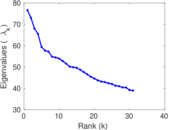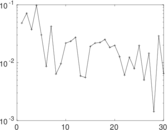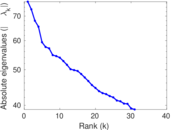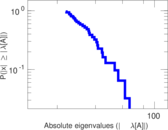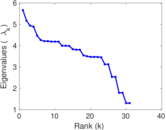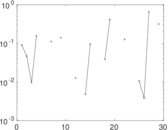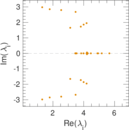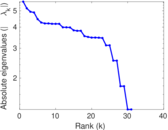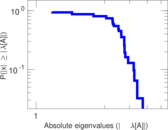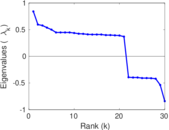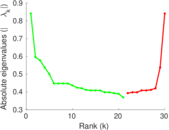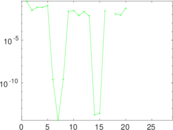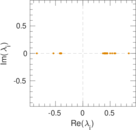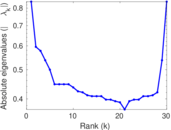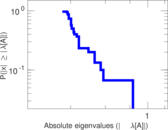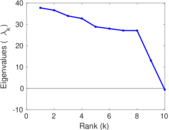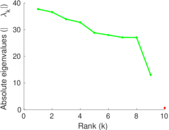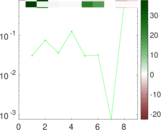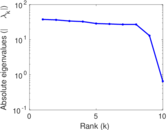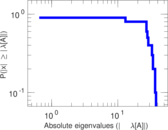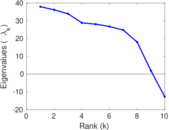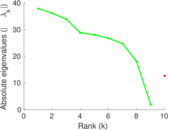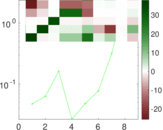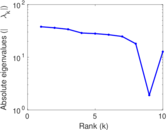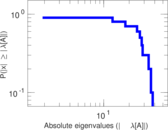# arXiv hep-ph

This is the network of publications in the arXiv's High Energy Physics – Phenomenology (hep-ph) section. The directed links that connect the publications are citations. Some publications cite themselves, and therefore the network contains loops.

 Code `PHc` Internal name `cit-HepPh` Name arXiv hep-ph Data source http://snap.stanford.edu/data/cit-HepPh.html AvailabilityDataset is available for download Consistency checkDataset passed all tests Category Citation network Node meaning Publication Edge meaning Citation Network format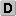Unipartite, directed Edge typeUnweighted, no multiple edges ReciprocalContains reciprocal edges Directed cyclesContains directed cycles Loops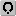Contains loops

## Statistics

 Size n = 34,546 Volume m = 421,578 Loop count l = 44 Wedge count s = 26,298,866 Claw count z = 1,344,423,338 Cross count x = 104,402,971,182 Triangle count t = 1,276,868 Square count q = 39,015,537 4-Tour count T4 = 418,161,514 Maximum degree dmax = 846 Maximum outdegree d+max = 411 Maximum indegree d−max = 846 Average degree d = 24.406 8 Fill p = 0.000 353 250 Size of LCC N = 34,401 Size of LSCC Ns = 12,711 Relative size of LSCC Nrs = 0.367 944 Diameter δ = 14 50-Percentile effective diameter δ0.5 = 3.817 19 90-Percentile effective diameter δ0.9 = 5.229 89 Median distance δM = 4 Mean distance δm = 4.395 35 Gini coefficient G = 0.525 062 Balanced inequality ratio P = 0.306 494 Outdegree balanced inequality ratio P+ = 0.311 838 Indegree balanced inequality ratio P− = 0.251 510 Relative edge distribution entropy Her = 0.952 172 Power law exponent γ = 1.378 28 Tail power law exponent γt = 3.041 00 Tail power law exponent with p γ3 = 3.041 00 p-value p = 0.000 00 Outdegree tail power law exponent with p γ3,o = 3.741 00 Outdegree p-value po = 0.150 000 Indegree tail power law exponent with p γ3,i = 3.071 00 Indegree p-value pi = 0.000 00 Degree assortativity ρ = −0.006 286 37 Degree assortativity p-value pρ = 8.040 38 × 10−9 In/outdegree correlation ρ± = +0.138 861 Clustering coefficient c = 0.145 657 Directed clustering coefficient c± = 0.196 720 Spectral norm α = 76.641 1 Operator 2-norm ν = 57.077 2 Cyclic eigenvalue π = 5.662 91 Algebraic connectivity a = 0.046 393 3 Spectral separation |λ1[A] / λ2[A]| = 1.049 45 Reciprocity y = 0.003 221 23 Non-bipartivity bA = 0.502 827 Normalized non-bipartivity bN = 0.057 397 4 Spectral bipartite frustration bK = 0.001 045 37 Controllability C = 8,745

## Plots

### Degree distribution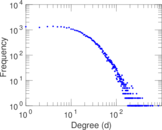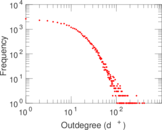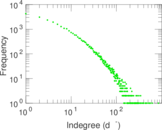### Cumulative degree distribution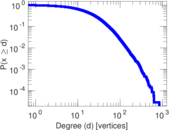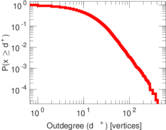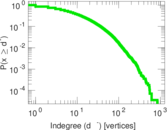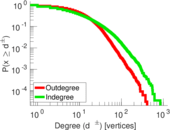### Lorenz curve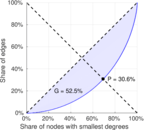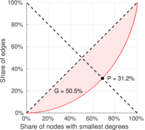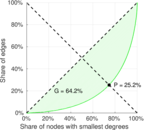### Spectral distribution of the adjacency matrix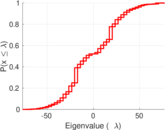### Spectral distribution of the normalized adjacency matrix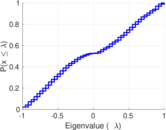### Spectral distribution of the Laplacian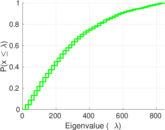### Spectral graph drawing based on the adjacency matrix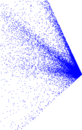### Spectral graph drawing based on the Laplacian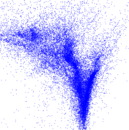### Spectral graph drawing based on the normalized adjacency matrix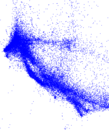### Degree assortativity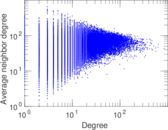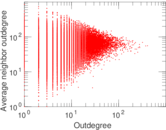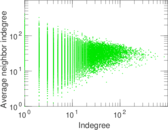### Zipf plot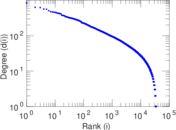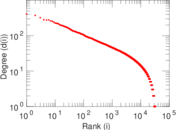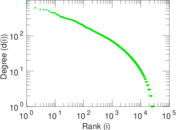### Hop distribution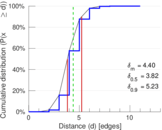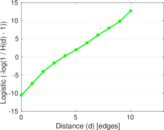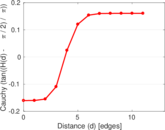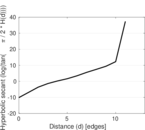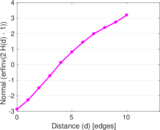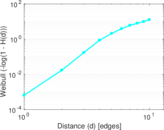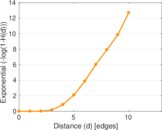### Delaunay graph drawing### In/outdegree scatter plot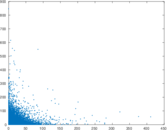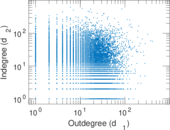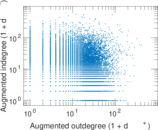### Clustering coefficient distribution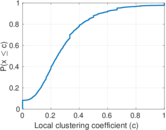### Average neighbor degree distribution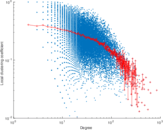### SynGraphy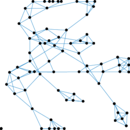### Matrix decompositions plots